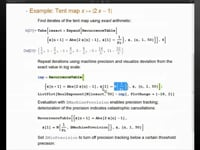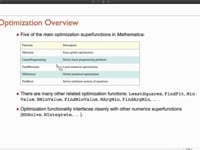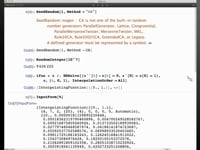Learn how »

# Solving Differential Equations in Mathematica

Get an overview of Mathematica's framework for solving differential equations in this presentation from Mathematica Experts Live: Numeric Modeling in Mathematica.